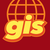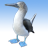# Getting X and Y from a single point object in arcpy

2010
6
Jump to solution
09-11-2018 01:56 PMOccasional Contributor

I am trying to get the X and Y values from the output of the positionAlongLine method.

As far as I can tell it should be a point object so how do I get the XY from that point?

I have found examples of how to do this in a search cursor but that only works with tables, I only have a single point.

`new_point = line.positionAlongLine(cur_length, is_percentage)point_x = new_point.Xpoint_y = new_point.Y`

returns the error: AttributeError: 'PointGeometry' object has no attribute 'X'

Any help would be greatly appreciated.

Tags (2)
1 Solution

Accepted SolutionsbyMVP Esteemed Contributor

Try

``new_point.centroid.X‍‍‍``

from here

6 RepliesbyMVP Esteemed Contributor

Try

``new_point.centroid.X‍‍‍``

from hereOccasional Contributor

Thank you very much Dan. That worked perfectly.byMVP Esteemed Contributor

No problem... it is one of those quirky things, but think of it as a property of the object... it helped me get over it a long time agobyEsri Esteemed Contributor

To add to the explanation by Dan Patterson here a little more about the difference between Point and PointGeometry objects. The Point object is used to store the X, Y, and optionally the Z and M values of a point. The Point object iis used to construct geometries, like PointGeometry, Polyline, Polygon and Multipoint. See example below:

``import arcpy# create a Point with just X and Y (Long and Lat)pnt = arcpy.Point(-75.569461, 6.216609)print("X (pnt) : {}".format(pnt.X))# create PointGeometry using WGS1984sr = arcpy.SpatialReference(4326)pntg = arcpy.PointGeometry(pnt, sr)# retrieve the X property of the PointGeometry (I normally use firstPoint)print("X (pntg): {}".format(pntg.firstPoint.X))‍‍‍‍‍‍‍‍‍‍‍‍``

This will print:

``X (pnt) : -75.569461X (pntg): -75.569461‍‍``

To construct the Polyline, Polygon and Multipoint geometries you will have to provide an array of Points. See example below:

``import arcpy# simple example, create two Points with just X and Y (Long and Lat)pnt1 = arcpy.Point(-75.569461, 6.216609)pnt2 = arcpy.Point(-74.051171, 4.673431)# create Polyline using WGS1984sr = arcpy.SpatialReference(4326)polyline = arcpy.Polyline(arcpy.Array([pnt1, pnt2]), sr)# retrieve some coordinate valuesprint("X (firstPoint): {}".format(polyline.firstPoint.X))print("Y (lastPoint) : {}".format(polyline.lastPoint.Y))# loop through pnts in Polylinefor part in polyline:    for pnt in part:        print(" - {}".format(pnt))‍‍‍‍‍‍‍‍‍‍‍‍‍‍‍‍‍‍``

When working with 3D and M aware geometries you will have to enable those properties when you construct the geometry. More on this in my blog here: https://community.esri.com/people/xander_bakker/blog/2016/07/08/working-with-3d-and-m-aware-geometri...byEsri Contributor

Can you use arcpy.da.searchcursor to get the x,y?byEsri Esteemed Contributor

Hi Liran Sun ,

Yes you can. The easiest way would be using the SHAPE@XY token. See:SearchCursor—Data Access module | Documentation